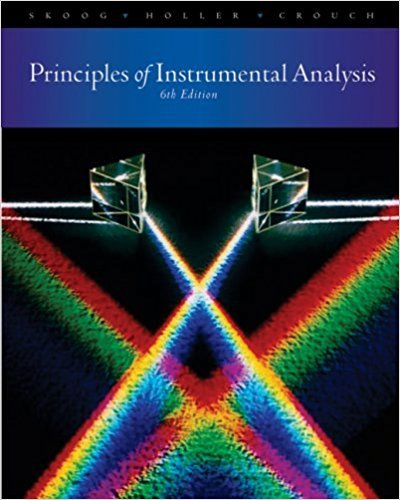×
×

# Solutions for Chapter 24: Coulometry## Full solutions for Principles of Instrumental Analysis | 6th Edition

ISBN: 9780495012016Solutions for Chapter 24: Coulometry

Solutions for Chapter 24
4 5 0 423 Reviews
13
1
##### ISBN: 9780495012016

Principles of Instrumental Analysis was written by and is associated to the ISBN: 9780495012016. This expansive textbook survival guide covers the following chapters and their solutions. Chapter 24: Coulometry includes 13 full step-by-step solutions. This textbook survival guide was created for the textbook: Principles of Instrumental Analysis , edition: 6. Since 13 problems in chapter 24: Coulometry have been answered, more than 13664 students have viewed full step-by-step solutions from this chapter.

Key Chemistry Terms and definitions covered in this textbook
• Allylic carbon

A carbon adjacent to a carbon-carbon double bond.

• Amino group

A compound containing an sp3 -hybridized nitrogen atom bonded to one, two, or three carbon atoms

• becquerel

The SI unit of radioactivity. It corresponds to one nuclear disintegration per second. (Section 21.4)

• Beer’s law

In UV-Vis spectroscopy, an equation describing the relationship between molar absorptivity (e), absorbance (A), concentration (C), and path length (l): e = A (C Ž l)

• boiling point.

The temperature at which the vapor pressure of a liquid is equal to the external atmospheric pressure. (11.8)

• combination reaction

A chemical reaction in which two or more substances combine to form a single product. (Section 3.2)

• coupling constant

When signal splitting occurs in NMR spectroscopy, the distance between the individual peaks of a signal.

• downfield

The left side of an NMR spectrum.

• epoxide

A cyclic ether containing a three-membered ring system. Also called an oxirane (also see Sect. 14.7).

• frequency

The number of times per second that one complete wavelength passes a given point. (Section 6.1)

• fuel cell

A voltaic cell that utilizes the oxidation of a conventional fuel, such as H2 or CH4, in the cell reaction. (Section 20.7)

• heterogeneous catalyst

A catalyst that does not dissolve in the reaction medium.

• homotopic

Protons that are interchangeable by rotational symmetry.

• hypothesis

A tentative explanation of a series of observations or of a natural law. (Section 1.3)

• mass-to-charge ratio(m/z)

Thedetermining factor by which ions are separatedfrom each other in mass spectrometry.

• N-glycoside

The product obtained when a monosaccharide is treated with an amine in the presence of an acid catalyst.

• overlap

The extent to which atomic orbitals on different atoms share the same region of space. When the overlap between two orbitals is large, a strong bond may be formed. (Section 9.4)

• Rate determining step

The step in a multistep reaction sequence that crosses the highest energy barrier.

• resonance structures

A series of structures that are melded together (conceptually) to circumvent the inadequacies of bond-line drawings.

• Sulfi de

The sulfur analog of an ether; a molecule containing a sulfur atom bonded to two carbon atoms. Sulfi des are also called thioethers

×